# PicoCTF 2018 - KeyGen 1 WriteUp

Here we are with a KeyGen challenge.

## KeyGen 1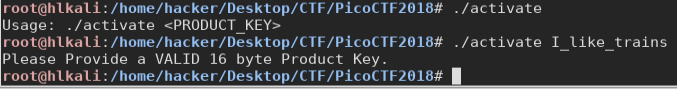In the main we can see 2 function: Check_valid_key and validate_key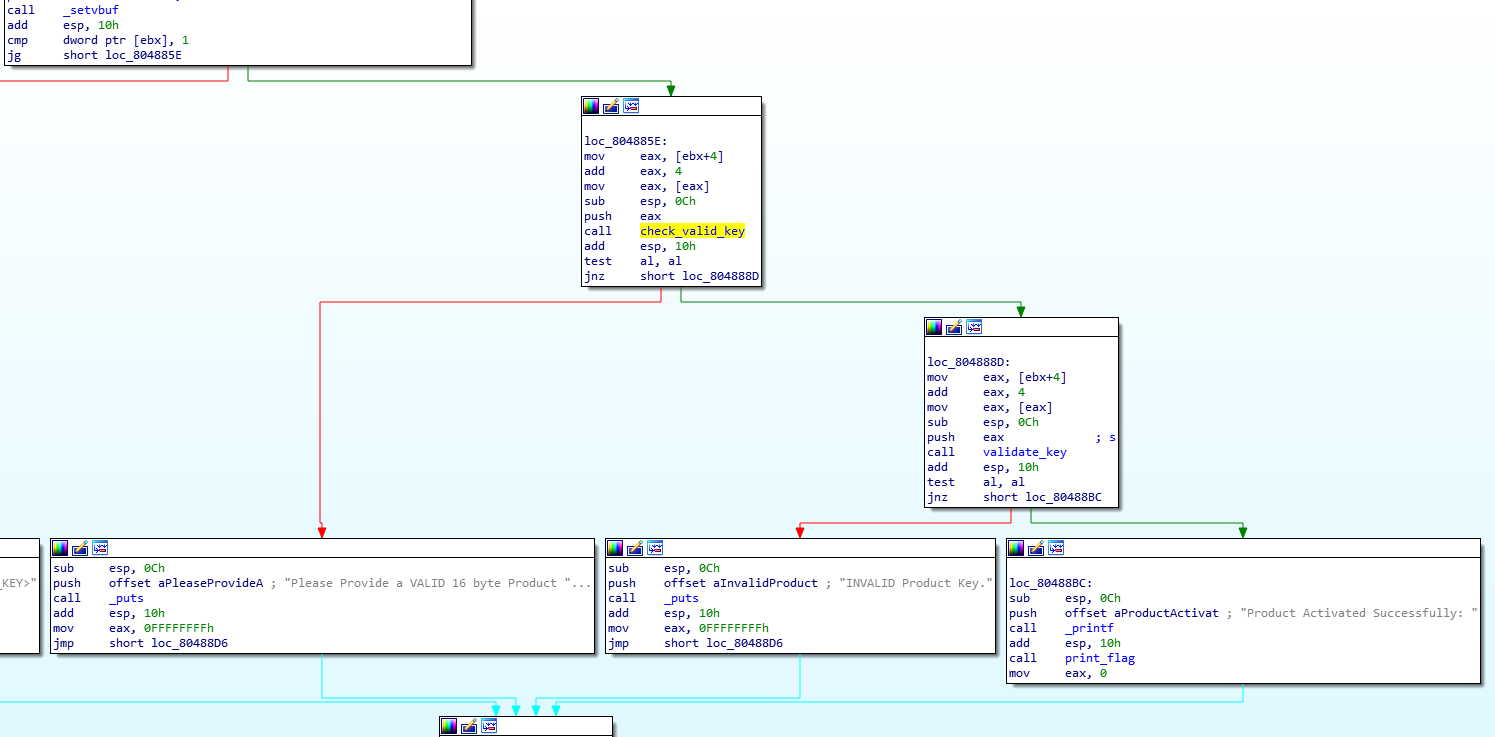Reading the output below, we can guess that the first check only if the input is of the right length and use some valid chars, the latter, checks more deeply if the whole key is correct.

Let’s see:

### check_valid_key

As I thought, on the right we see a for loop (index is var_5) that count (var_4) the length of the string.

It has to be 16.
In the middle, we see that a function “check_valid_char” is called.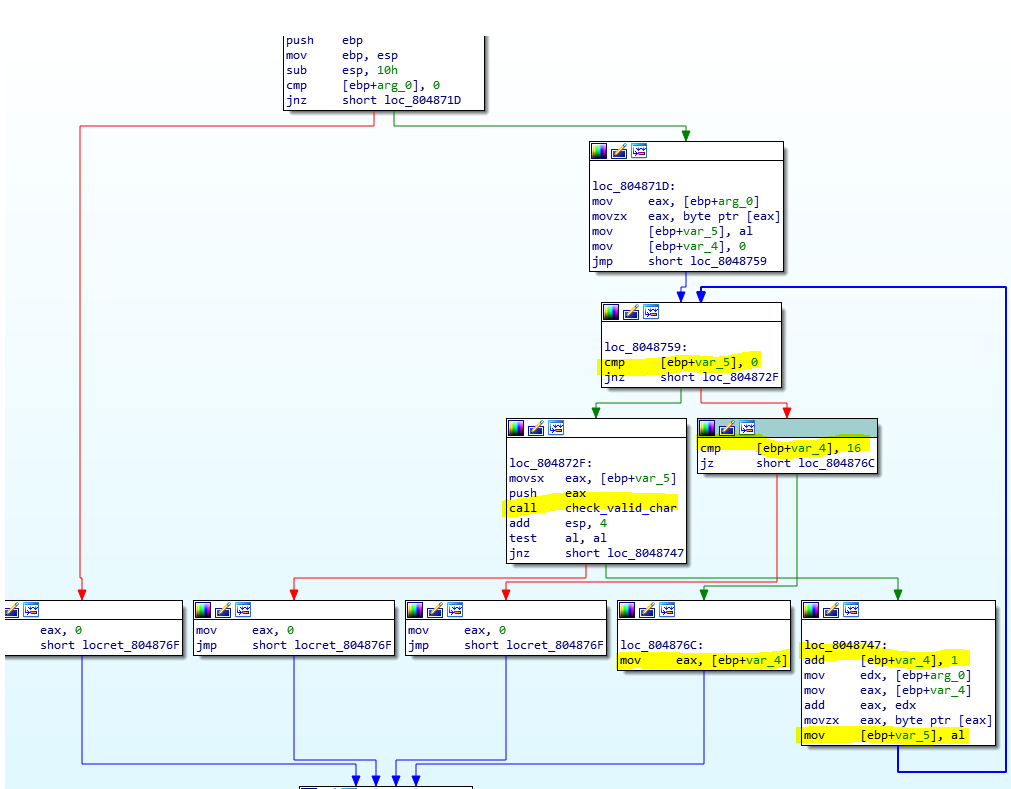### check_valid_char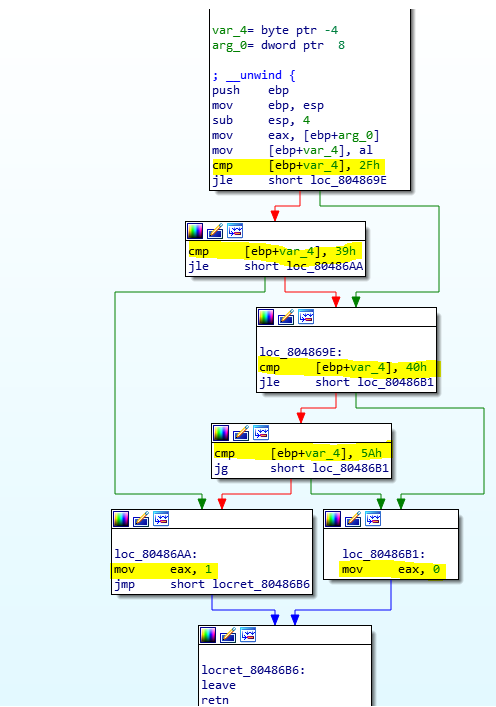As we can see our key can contains only chars in those ranges (0:9 and A: Z).

Now we know what chars are allowed, let’s move back to the main, and in the second function.

### validate_key

We see here a loop on the length of the key, a function “ord” is called, and something is “added” each time in var_14 (set to 0 before the loop).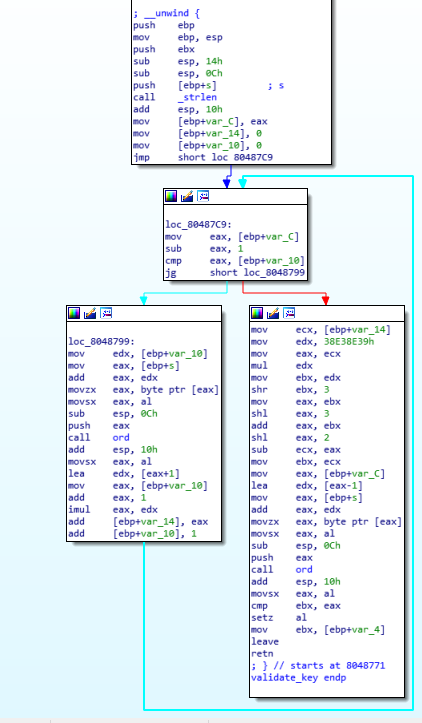On the right side, after the loop, we see that ord is called again, using as argument the last char of the key, a comparison between ebx (the value accumulated in the loop after some manipulation) and eax is performed, if they are equal, it returns ‘1’ which means that the key is correct.

The tricky part is to notice that “some manipulation” is actually a modulo operation, infact multiply by 38E38E39h and shift rotates right 3 bits resoults in division by 36. (Noticed debugging it with gdb).

I also found this:
http://www.hackersdelight.org/magic.htm
which is a tool that gives you the “magic numbers” to perform a division by multiply and shifting.

### One more thing to check, ord function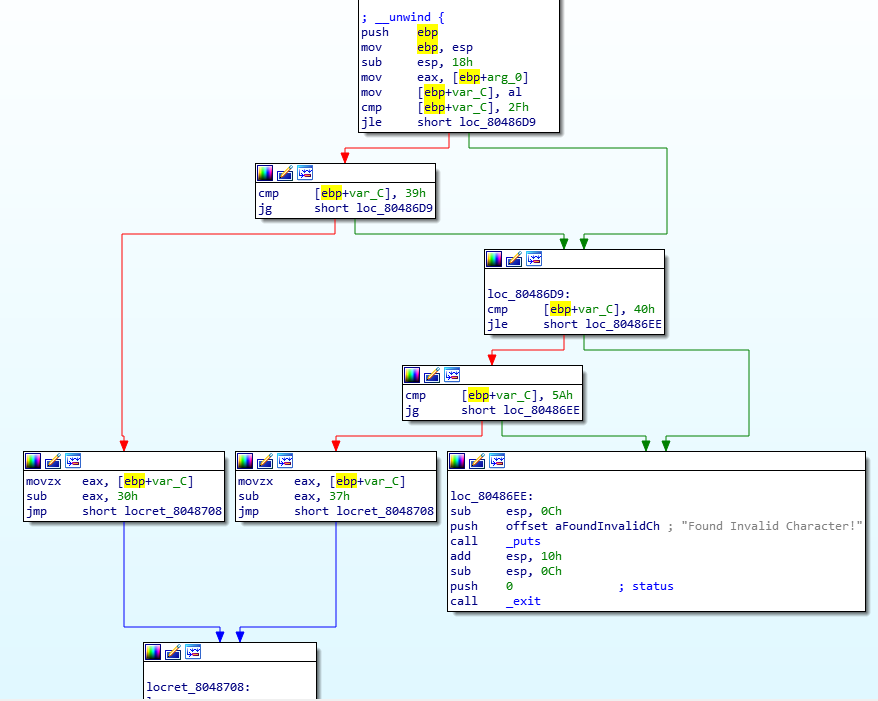Thankfully it is quite simple:

## Let’s put ideas together

Okay, here what’s happening:
The key is read, every char is checked to be good (0:9 and A:Z)
next in “validate_key” function a check like this is performed:

## Okay but what is the vulnerability here?

Thanks to the fact that to the ‘acc’ variable is performed a modulo operation, we know that it’s value is from 0 to 35.
Since the verify function simply “accumulate” the value of chars (after some minor transformation performed by ord) we can fairly say that the probability of finding a good key choosing by random chars (and using the correct charset) is 1/36.

So even if it is a 16 chars password, we can try to brute-force it.

Here’s the code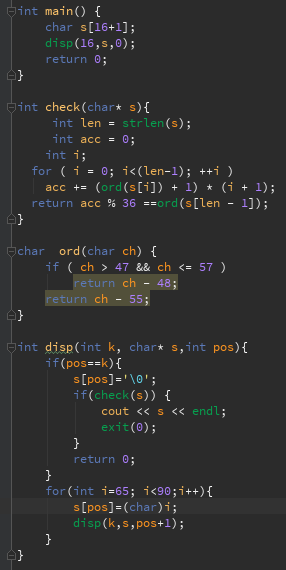and it produce:
AAAAAAAAAAAAAAAO

That’s it!

XxcoralloxX